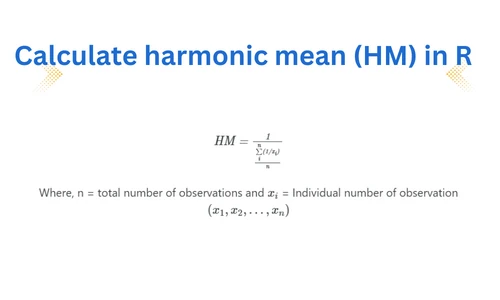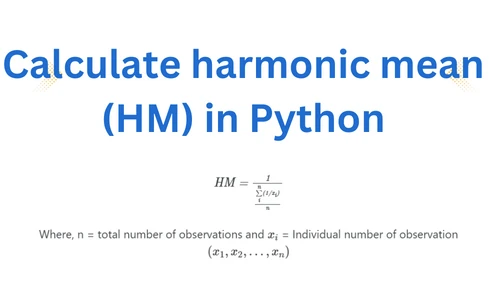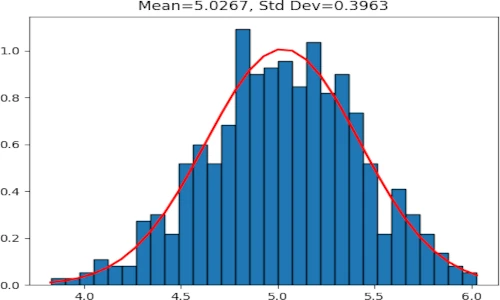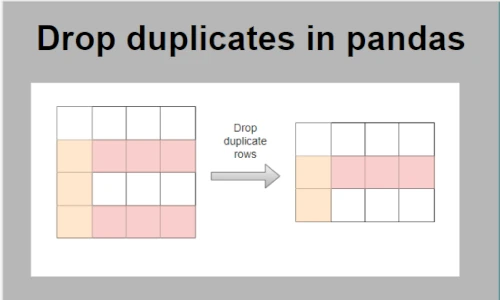# Make discoveries with your data

## Subscribe to get new article to your email when published

* indicates required

## Data handling and manipulation with Pandas

What is pandas?## How to replace column values in pandas DataFrame based on column conditions

Learn four methods to replace the column values in pandas DataFrame based on column conditions## How to unpivot pandas DataFrame (wide to long format)

Learn how to convert pandas DataFrame from wide to long format using melt() function

## Pandas groupby function to group column values into list

Group dataframe rows into a list based on a common element from one column## How to split strings into a list of integers in Python?

Learn how to split the strings as an int list in Python## How to multiply two or multiple columns in a pandas DataFrame

Learn how to multiply single or multiple columns in a pandas DataFrame## Calculate harmonic mean in R (with examples)

Learn what is harmonic mean and how to calculate it in R## Calculate harmonic mean in Python (with examples)

Learn what is harmonic mean and how to calculate it in Python## Generate random dataset with normal distribution in Python (using NumPy)

Learn how to generate a random dataset with normal distribution using NumPy